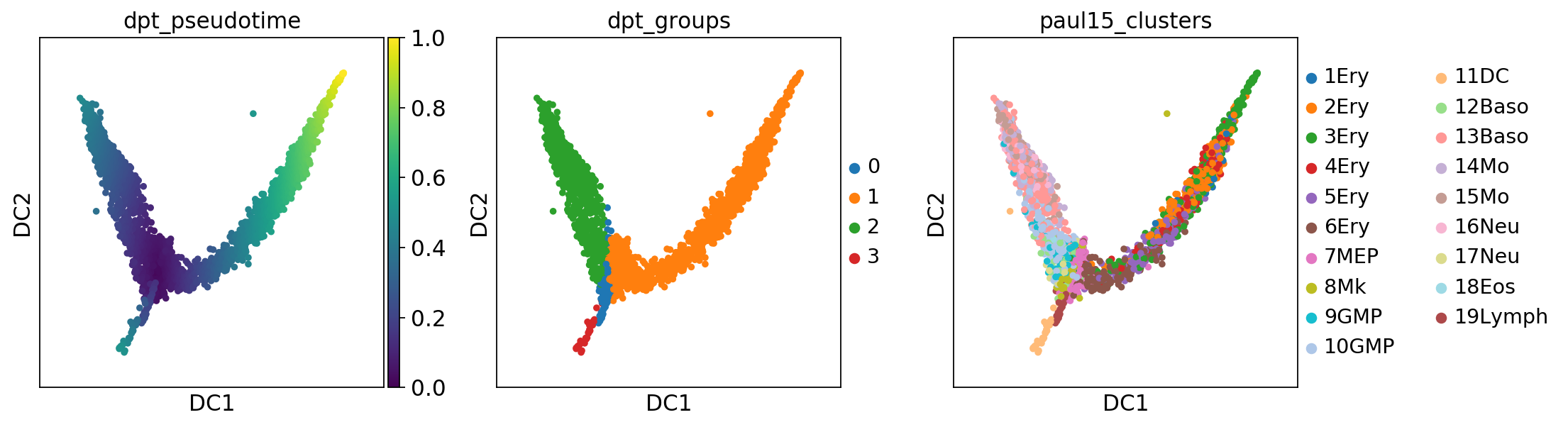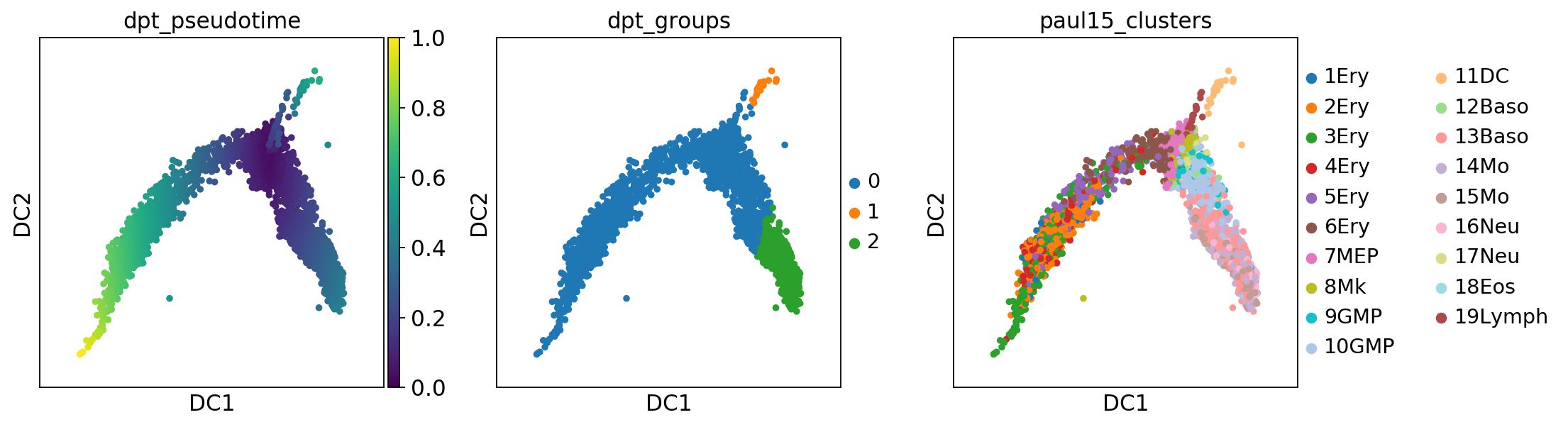# DPT for hematopoiesis in mouse Paul et al., Cell (2015)¶

Hematopoiesis: trace myeloid and erythroid differentiation for data of Paul et al., Cell (2015).

Note: For a more recent and richer analysis of this dataset take a look at https://github.com/theislab/graph_abstraction/tree/master/paul15.

In :
import numpy as np
import matplotlib.pyplot as pl
import scanpy.api as sc

sc.settings.verbosity = 3  # verbosity: errors (0), warnings (1), info (2), hints (3)
sc.logging.print_versions()

scanpy==1.3.1+83.g641af42 anndata==0.6.10+31.g4c40622 numpy==1.14.5 scipy==1.1.0 pandas==0.23.4 scikit-learn==0.19.1 statsmodels==0.9.0 python-igraph==0.7.1 louvain==0.6.1

In :
sc.settings.set_figure_params(dpi=80)  # low dpi (dots per inch) yields small inline figures


Perform a simple Diffusion Pseudotime analysis on raw data, as in Haghverdi et al. (2016). No preprocessing, only logarthmize the raw counts.

Note: The following function is also available as sc.datasets.paul15().

In :
adata = sc.datasets.paul15()

... storing 'paul15_clusters' as categorical

WARNING: In Scanpy 0.*, this returned logarithmized data. Now it returns non-logarithmized data.

In :
sc.pp.log1p(adata)  # logarithmize data

In :
sc.pp.neighbors(adata, n_neighbors=20, use_rep='X', method='gauss')

computing neighbors
finished (0:00:01.95) --> added to .uns['neighbors']
'distances', weighted adjacency matrix
'connectivities', weighted adjacency matrix

In :
sc.tl.diffmap(adata)

computing Diffusion Maps using n_comps=15(=n_dcs)
eigenvalues of transition matrix
[1.         0.992215   0.966011   0.9404994  0.9273089  0.88551635
0.8672743  0.8413333  0.82867014 0.8070438  0.76248974 0.7282334
0.7271825  0.7115725  0.69101715]
finished (0:00:00.05) --> added
'X_diffmap', diffmap coordinates (adata.obsm)
'diffmap_evals', eigenvalues of transition matrix (adata.uns)

In :
sc.tl.dpt(adata, n_branchings=1, n_dcs=10)

computing Diffusion Pseudotime using n_dcs=10
finished (0:00:00.61) --> added
'dpt_pseudotime', the pseudotime (adata.obs)
'dpt_groups', the branching subgroups of dpt (adata.obs)
'dpt_order', cell order (adata.obs)


Diffusion Pseudotime (DPT) analysis detects the branch of granulocyte/macrophage progenitors (GMP), and the branch of megakaryocyte/erythrocyte progenitors (MEP). There are two small further subgroups (segments 0 and 2).

In :
sc.pl.diffmap(adata, color=['dpt_pseudotime', 'dpt_groups', 'paul15_clusters'])With this, we reproduced the analysis of Haghverdi et al. (2016, Suppl. Note 4 and Suppl. Figure N4).

In :
adata.write(results_file)


Computing connectivities using UMAP gives us quantitiatively different results for the pseudotime.

In :
sc.pp.neighbors(adata, n_neighbors=20, use_rep='X', method='umap')

computing neighbors
finished (0:00:05.29) --> added to .uns['neighbors']
'distances', weighted adjacency matrix
'connectivities', weighted adjacency matrix

In :
sc.tl.diffmap(adata)

computing Diffusion Maps using n_comps=15(=n_dcs)
eigenvalues of transition matrix
[1.         0.993715   0.9717146  0.95699656 0.94785434 0.9100064
0.89768046 0.8868277  0.8667819  0.8511887  0.82064795 0.80687267
0.7805664  0.77649134 0.7696744 ]
finished (0:00:00.05) --> added
'X_diffmap', diffmap coordinates (adata.obsm)
'diffmap_evals', eigenvalues of transition matrix (adata.uns)

In :
sc.tl.dpt(adata, n_branchings=1)

computing Diffusion Pseudotime using n_dcs=10
finished (0:00:00.63) --> added
'dpt_pseudotime', the pseudotime (adata.obs)
'dpt_groups', the branching subgroups of dpt (adata.obs)
'dpt_order', cell order (adata.obs)

In :
sc.pl.diffmap(adata, color=['dpt_pseudotime', 'dpt_groups', 'paul15_clusters'])